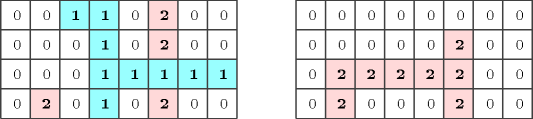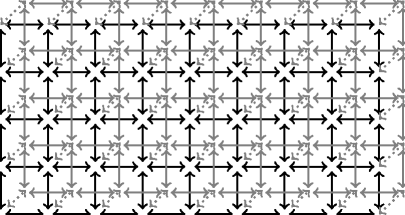## 5.85. connect_points

Origin

N. Beldiceanu

Constraint

$\mathrm{𝚌𝚘𝚗𝚗𝚎𝚌𝚝}_\mathrm{𝚙𝚘𝚒𝚗𝚝𝚜}\left(\mathrm{𝚂𝙸𝚉𝙴}\mathtt{1},\mathrm{𝚂𝙸𝚉𝙴}\mathtt{2},\mathrm{𝚂𝙸𝚉𝙴}\mathtt{3},\mathrm{𝙽𝙶𝚁𝙾𝚄𝙿},\mathrm{𝙿𝙾𝙸𝙽𝚃𝚂}\right)$

Arguments
 $\mathrm{𝚂𝙸𝚉𝙴}\mathtt{1}$ $\mathrm{𝚒𝚗𝚝}$ $\mathrm{𝚂𝙸𝚉𝙴}\mathtt{2}$ $\mathrm{𝚒𝚗𝚝}$ $\mathrm{𝚂𝙸𝚉𝙴}\mathtt{3}$ $\mathrm{𝚒𝚗𝚝}$ $\mathrm{𝙽𝙶𝚁𝙾𝚄𝙿}$ $\mathrm{𝚍𝚟𝚊𝚛}$ $\mathrm{𝙿𝙾𝙸𝙽𝚃𝚂}$ $\mathrm{𝚌𝚘𝚕𝚕𝚎𝚌𝚝𝚒𝚘𝚗}\left(𝚙-\mathrm{𝚍𝚟𝚊𝚛}\right)$
Restrictions
 $\mathrm{𝚂𝙸𝚉𝙴}\mathtt{1}>0$ $\mathrm{𝚂𝙸𝚉𝙴}\mathtt{2}>0$ $\mathrm{𝚂𝙸𝚉𝙴}\mathtt{3}>0$ $\mathrm{𝙽𝙶𝚁𝙾𝚄𝙿}\ge 0$ $\mathrm{𝙽𝙶𝚁𝙾𝚄𝙿}\le |\mathrm{𝙿𝙾𝙸𝙽𝚃𝚂}|$ $\mathrm{𝚂𝙸𝚉𝙴}\mathtt{1}*\mathrm{𝚂𝙸𝚉𝙴}\mathtt{2}*\mathrm{𝚂𝙸𝚉𝙴}\mathtt{3}=|\mathrm{𝙿𝙾𝙸𝙽𝚃𝚂}|$ $\mathrm{𝚛𝚎𝚚𝚞𝚒𝚛𝚎𝚍}$$\left(\mathrm{𝙿𝙾𝙸𝙽𝚃𝚂},𝚙\right)$
Purpose

On a 3-dimensional grid of variables, number of groups, where a group consists of a connected set of variables that all have a same value distinct from 0.

Example
$\left(\begin{array}{c}8,4,2,2,〈\begin{array}{c}𝚙-0,𝚙-0,\hfill \\ 𝚙-1,𝚙-1,\hfill \\ 𝚙-0,𝚙-2,\hfill \\ 𝚙-0,𝚙-0,\hfill \\ 𝚙-0,𝚙-0,\hfill \\ 𝚙-0,𝚙-1,\hfill \\ 𝚙-0,𝚙-2,\hfill \\ 𝚙-0,𝚙-0,\hfill \\ 𝚙-0,𝚙-0,\hfill \\ 𝚙-0,𝚙-1,\hfill \\ 𝚙-1,𝚙-1,\hfill \\ 𝚙-1,𝚙-1,\hfill \\ 𝚙-0,𝚙-2,\hfill \\ 𝚙-0,𝚙-1,\hfill \\ 𝚙-0,𝚙-2,\hfill \\ 𝚙-0,𝚙-0,\hfill \\ 𝚙-0,𝚙-0,\hfill \\ 𝚙-0,𝚙-0,\hfill \\ 𝚙-0,𝚙-0,\hfill \\ 𝚙-0,𝚙-0,\hfill \\ 𝚙-0,𝚙-0,\hfill \\ 𝚙-0,𝚙-0,\hfill \\ 𝚙-0,𝚙-2,\hfill \\ 𝚙-0,𝚙-0,\hfill \\ 𝚙-0,𝚙-2,\hfill \\ 𝚙-2,𝚙-2,\hfill \\ 𝚙-2,𝚙-2,\hfill \\ 𝚙-0,𝚙-0,\hfill \\ 𝚙-0,𝚙-2,\hfill \\ 𝚙-0,𝚙-0,\hfill \\ 𝚙-0,𝚙-2,\hfill \\ 𝚙-0,𝚙-0\hfill \end{array}〉\hfill \end{array}\right)$

Figure 5.85.1 corresponds to the solution where we describe separately each layer of the grid. The $\mathrm{𝚌𝚘𝚗𝚗𝚎𝚌𝚝}_\mathrm{𝚙𝚘𝚒𝚗𝚝𝚜}$ constraint holds since we have two groups ($\mathrm{𝙽𝙶𝚁𝙾𝚄𝙿}=2$): a first one for the variables of the $\mathrm{𝙿𝙾𝙸𝙽𝚃𝚂}$ collection assigned to value 1, and a second one for the variables assigned to value 2.

##### Figure 5.85.1. The two layers of the solutionTypical
 $\mathrm{𝚂𝙸𝚉𝙴}\mathtt{1}>1$ $\mathrm{𝚂𝙸𝚉𝙴}\mathtt{2}>1$ $\mathrm{𝙽𝙶𝚁𝙾𝚄𝙿}>0$ $\mathrm{𝙽𝙶𝚁𝙾𝚄𝙿}<|\mathrm{𝙿𝙾𝙸𝙽𝚃𝚂}|$ $|\mathrm{𝙿𝙾𝙸𝙽𝚃𝚂}|>3$
Symmetry

All occurrences of two distinct values of $\mathrm{𝙿𝙾𝙸𝙽𝚃𝚂}.𝚙$ that are both different from 0 can be swapped; all occurrences of a value of $\mathrm{𝙿𝙾𝙸𝙽𝚃𝚂}.𝚙$ that is different from 0 can be renamed to any unused value that is also different from 0.

Arg. properties

Functional dependency: $\mathrm{𝙽𝙶𝚁𝙾𝚄𝙿}$ determined by $\mathrm{𝚂𝙸𝚉𝙴}\mathtt{1}$, $\mathrm{𝚂𝙸𝚉𝙴}\mathtt{2}$, $\mathrm{𝚂𝙸𝚉𝙴}\mathtt{3}$ and $\mathrm{𝙿𝙾𝙸𝙽𝚃𝚂}$.

Usage

Wiring problems [Simonis90][Zhou96].

Algorithm

Since the graph corresponding to the 3-dimensional grid is symmetric one could certainly use as a starting point the filtering algorithm associated with the number of connected components graph property described in [BeldiceanuPetitRochart06] (see the paragraphs “Estimating $\underline{\mathrm{𝐍𝐂𝐂}}$” and “Estimating $\overline{\mathrm{𝐍𝐂𝐂}}$”). One may also try to take advantage of the fact that the considered initial graph is a grid in order to simplify the previous filtering algorithm.

Keywords
Arc input(s)

$\mathrm{𝙿𝙾𝙸𝙽𝚃𝚂}$

Arc generator
$\mathrm{𝐺𝑅𝐼𝐷}$$\left(\left[\mathrm{𝚂𝙸𝚉𝙴}\mathtt{1},\mathrm{𝚂𝙸𝚉𝙴}\mathtt{2},\mathrm{𝚂𝙸𝚉𝙴}\mathtt{3}\right]\right)↦\mathrm{𝚌𝚘𝚕𝚕𝚎𝚌𝚝𝚒𝚘𝚗}\left(\mathrm{𝚙𝚘𝚒𝚗𝚝𝚜}\mathtt{1},\mathrm{𝚙𝚘𝚒𝚗𝚝𝚜}\mathtt{2}\right)$

Arc arity
Arc constraint(s)
 $•\mathrm{𝚙𝚘𝚒𝚗𝚝𝚜}\mathtt{1}.𝚙\ne 0$ $•\mathrm{𝚙𝚘𝚒𝚗𝚝𝚜}\mathtt{1}.𝚙=\mathrm{𝚙𝚘𝚒𝚗𝚝𝚜}\mathtt{2}.𝚙$
Graph property(ies)
$\mathrm{𝐍𝐒𝐂𝐂}$$=\mathrm{𝙽𝙶𝚁𝙾𝚄𝙿}$

Graph class
$\mathrm{𝚂𝚈𝙼𝙼𝙴𝚃𝚁𝙸𝙲}$

Graph model

Figure 5.85.2 gives the initial graph constructed by the $\mathrm{𝐺𝑅𝐼𝐷}$ arc generator associated with the Example slot.

##### Figure 5.85.2. Graph generated by $\mathrm{𝐺𝑅𝐼𝐷}$([8,4,2])